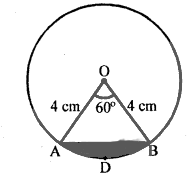# $A B$ is a chord of a circle with centre $O$ and radius $4 \mathrm{~cm} . A B$ is of length $4 \mathrm{~cm}$ and divides the circle into two segments. Find the area of the minor segment.

Given:

$A B$ is a chord of a circle with centre $O$ and radius $4 \mathrm{~cm} . A B$ is of length $4 \mathrm{~cm}$ and divides the circle into two segments.

To do:

We have to find the area of the minor segment.

Solution:Radius of the circle $r = 4\ cm$
Length of the chord $AB = 4\ cm$

In $\triangle OAB$,
$OA = OB = AB = 4\ cm$

This implies,

$\Delta \mathrm{OAB}$ is an equilateral triangle.

Therefore,

$\angle \mathrm{AOB}=\theta=60^{\circ}$

Area of the minor segment $\mathrm{ADB}=(\frac{\pi \theta}{360^{\circ}}-\sin \frac{\theta}{2} \cos \frac{\theta}{2}) r^{2}$

$=(\frac{\pi \times 60^{\circ}}{360^{\circ}}-\sin \frac{60^{\circ}}{2} \cos \frac{60^{\circ}}{2})(4)^{2}$

$=(\frac{\pi}{6}-\sin 30^{\circ} \cos 30^{\circ}) \times 16$

$=16(\frac{\pi}{6}-\frac{1}{2} \times \frac{\sqrt{3}}{2})$

$=16(\frac{\pi}{6}-\frac{\sqrt{3}}{4})$

$=(\frac{16 \times \pi}{6}-16 \times \frac{\sqrt{3}}{4})$

$=(\frac{8 \pi}{3}-4 \sqrt{3}) \mathrm{cm}^{2}$

The area of the minor segment is $(\frac{8 \pi}{3}-4 \sqrt{3}) \mathrm{cm}^{2}$.

Updated on: 10-Oct-2022

26 Views